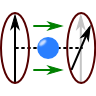## The Faraday Rotation - How an Electron Gas and a Magnetic Field Rotate a Plane WaveIn The Dispersion Relation of a Magnetized Plasma we found that a magnetic field alters the dispersion relation differently for left and right polarized light passing through a plasma. But what about a linear polarized wave? The emerging effect is termed the "Faraday Rotation" and has numerous applications. Find out what we can learn about the cosmos and graphene using this effect.

##Problem Statement

In the mentioned problem we assumed an electron density $$n_{e}$$ and a constant magnetic field $$\mathbf{B}=B_{0}\mathbf{e}_{z}$$. We wanted to know the dispersion relation for circularly polarized light, $\mathbf{E}^{l/r}\left(\mathbf{r},t\right) = E_{0}\exp\left(\mathrm{i}\left(kz-\omega t\right)\right)\left[\mathbf{e}_{x}\pm\mathrm{i}\mathbf{e}_{y}\right]\ .$We found that $\epsilon_{r}^{l/r}\left(\omega\right) = 1-\frac{\omega_{p}^{2}}{\omega\left(\omega\mp\Omega\right)}$with the plasma and cylcotron frequencies $\omega_{p}^{2}=\frac{n_{e}e^{2}}{\epsilon_{0}m_{e}} , \Omega=eB_{0}/m_{e}\ .$In conclusion the response of the electron plasma was strongly depending on the polarization of the wave.

Find the rotation of a plane wave polarized in $$x$$ direction passing through a magnetized plasma of thickness $$d$$  for frequencies much greater than both $$\omega_{p}$$ and $$\Omega$$!

## Background: The Faraday Effect and its Applications

It is clear that any time more or less free charged particles are passed by an electromagnetic wave and a magnetic field is involved, one might observe the Faraday Effect we are about to calculate. One tool designed from the effect is the Faraday Rotator changing the angle of polarization due to the studied effect.

If one knows the rotation and the plasma density, one can in principle measure the magnetic field. This is also true for the biggest laboratory one can imagine - the universe! See for example Faraday Rotation of Microwave Background Polarization by a Primordial Magnetic Field on how the effect is used to unravel the last secrets of space and time.

We might very well change the length scales from lightyears to Ångström. Here we find the very promising material graphene which is a monolayer of carbon atoms. Graphene has an extremely high conductivity because of hybridized electrons. Thus, when a light wave is passing through this approximately five Ångström thick layer one might still expect a measurable Faraday Rotation. An experiment was done in 2010 and show the extraordinary properties of graphene, see Giant Faraday rotation in single- and multilayer graphene.